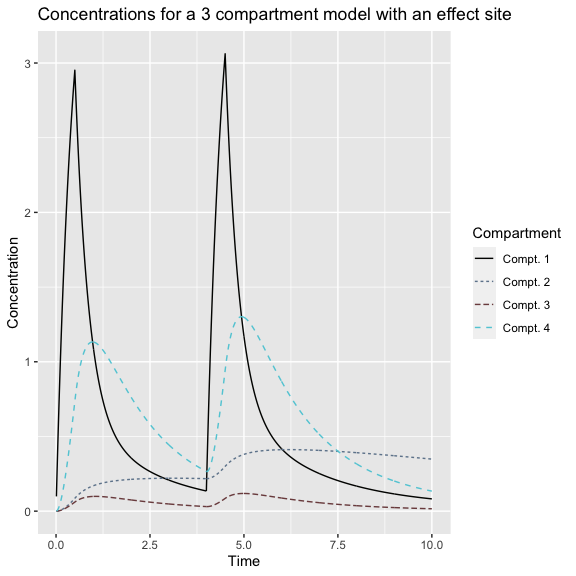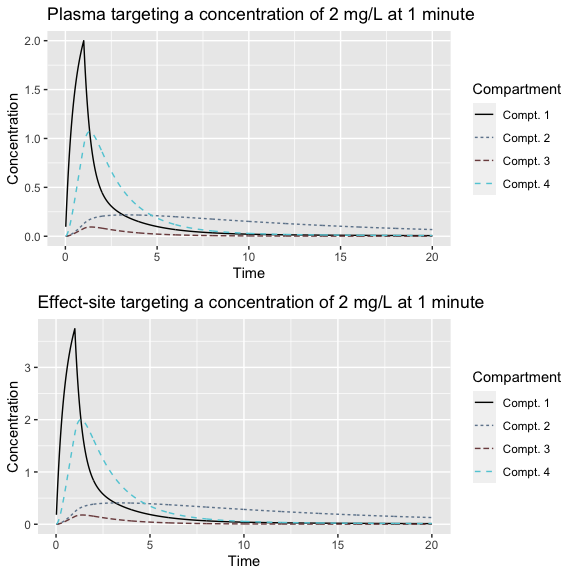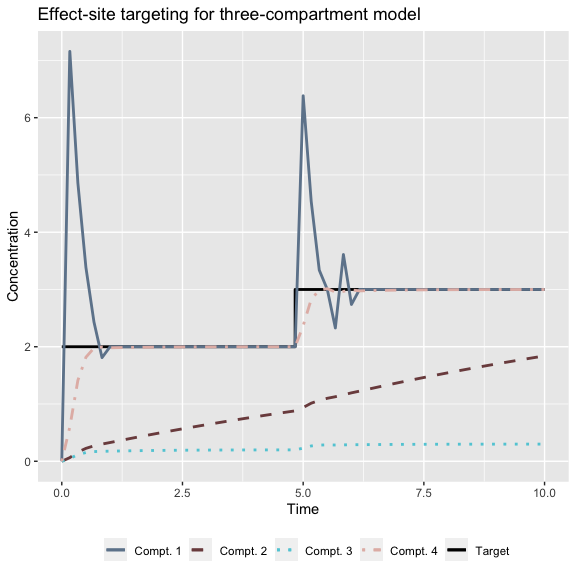# Introduction to tci package

## Overview

The tci package implements target-controlled infusion (TCI) algorithms for intravenous drugs with pharmacokinetics (PK) described by compartmental ordinary differential equation (ODE) based models. TCI systems are used in open- or closed-loop settings to partially or fully automate the delivery of medication to a patient, respectively. For a given patient, a pharmacokinetic or pharmacokinetic-pharmacodynamic (PK-PD) model is identified that describes the patient’s predicted response to the medication. This model is inverted by a TCI algorithm to supply the user (i.e. clinician) with infusion rates and durations that are expected to achieve a desired concentration or effect in the patient.

Within the tci package, users can select the Jacobs (1990) algorithm for plasma-targeting, the Shafer and Gregg (1992) algorithm for effect-site targeting, or define a custom TCI algorithm. Calculations are made using closed-form solutions to 1-, 2-, and 3-compartment PK models, as well as 3-compartment models with a fourth effect-site compartment. PK model code is based on solutions and code published by Abuhelwa, Foster, and Upton (2015). PK models based on other packages, such as mrgsolve, PKPDsim, and RxODE can be adapted for use within tci and are illustrated in a separate vignette. When an invertible PD model is specified, the algorithms in tci can be extended to PD outcomes.

Finally, tci provides a number of functions for simulating responses from patients receiving medications under open- or closed-loop TCI system administration. Data can be simulated assuming normal or log-normally distributed errors that are additive or multiplicative with respect to the response model. Closed-loop control is implemented using Bayesian updates to a patient-specific prior model. Use of these functions is demonstrated in a separate vignette. S3 plotting methods are additionally provided for visualizing dosing regimes and patient responses.

## Examples

library(tci)
library(knitr)
library(gridExtra)

### PK Models

For illustration of tci functions, we use a three compartment model with a fourth effect-site compartment. In reality, this is a four-compartment model; however, the fourth compartment is assumed to have negligible volume, such that its presence doesn’t interfere with the three-compartmental dynamics. This is achieved by setting the volume of the fourth compartment to be a minuscule fraction of the central compartment volume and setting $$k_{1e} = k_{e0}$$.(Shafer and Gregg 1992) The system of equations describing the three-compartment, effect-site model are below.

\begin{align} \dot{C}(t) = A*C(t) + B*k_R(t) \end{align}

\begin{align} A = \begin{pmatrix} -(k_{10}+k_{12}+k_{13}) & \frac{V_2}{V_1}k_{21} & \frac{V_3}{V_1}k_{31} & 0\\ \frac{V_1}{V_2}k_{12} & -k_{21} & 0 & 0 \\ \frac{V_1}{V_3}k_{13} & 0 & -k_{31} & 0 \\ k_{e0}/10^5 & 0 & 0 & -k_{e0} \end{pmatrix} \end{align}

\begin{align} B = [1,0,0,0]^T \end{align}

Infusion schedules must be passed alongside PK functions and are either created directly by the user or as the output of other tci functions described later. Infusion schedules must contain column names “infrt,” “begin,” and “end” designating to infusion rates with beginning and end times. The create_intvl function can be used to expand doses in the correct format by specifying infusion rates and end times with a start time that defaults to zero.

# e.g. infusion rates of 100 ug/min for 30 sec intervals at 0,4 minutes
dose <- create_intvl(
as.matrix(cbind(time = c(0.5,4,4.5,10),
infrt = c(100,0,100,0))),
inittm = 0
)
dose
#>      infrt begin  end
#> [1,]   100   0.0  0.5
#> [2,]     0   0.5  4.0
#> [3,]   100   4.0  4.5
#> [4,]     0   4.5 10.0

PK models are defined to have S3 class “pkmod” and can be evaluated through the predict_pkmod function in combination with a dosing schedule. Evaluation of a model additionally requires a set of PK model parameters. Starting concentrations, if unspecified, will be set to zero in each compartment. The units of measurement used for infusion rates and concentrations are not inherent to any structural PK model and may change depending on the drug and application. For the examples below, we will consider the intravenous anesthetic propofol, which is commonly described by a three-compartment model and used in TCI applications. Population models of propofol describe volumes in terms of liters (L), clearance parameters in terms of milliliters per minute (mL/min), and amounts of drug in terms of milligrams (mg). The infusion rate describes the quantity of propofol administered per unit of time on the same scale as the rest of the parameters in the PK model, and is therefore given in terms of mg/min.

# model parameters
pars_3cpt <- c(k10=1.5,k12=0.15,k21=0.09,k13=0.8,
k31=0.8,v1=10,v2=15,v3=100,ke0=1)

# predict concentrations of a three-compartment model with effect-site at
# times 1, 2, 8 minutes
predict_pkmod(pkmod3cptm,
pars = pars_3cpt,
inf = dose,
tms = c(1,2,8))
#>      time        c1        c2         c3        c4
#> [1,]    1 1.0812467 0.1708101 0.09872216 1.1317615
#> [2,]    2 0.3558635 0.2129614 0.07501638 0.7619403
#> [3,]    8 0.1675395 0.3914383 0.03653374 0.3157391

The same arguments can similarly be passed to a plot.pkmod method to display the patient responses over time.

# plot concentrations
plot(pkmod3cptm, inf = dose, pars = pars_3cpt,
title = "Concentrations for a 3 compartment model with an effect site")### TCI algorithms

The tci_plasma and tci_effect functions implement plasma and effect-site targeting, respectively. Each takes as arguments, a target concentration, Cpt, an infusion duration, dt, a pkmod class function, and PK model parameters. The output of each function is a single infusion rate that, if administered for dt, will result in the target concentrations at time dt for a patient with PK described by the specified model. Initial concentrations can optionally be passed as well through the argument “init.” Letting dt be expressed in terms of minutes, we can calculate the infusion rate (mg/min) required to reach a target concentration of 2 mg/L for a one-minute infusion.

# calculate infusion rates
kR_plasma <- tci_plasma(Cpt = 2, # target plasma concentration
dt = 1, # duration of infusion
pkmod = pkmod3cptm, # pk model
pars = pars_3cpt) # pk model parameters
kR_plasma
#>  49.58785

kR_effect <- tci_effect(Cet = 2, dt = 1, pkmod = pkmod3cptm, pars = pars_3cpt)

# set up dosing schedules
inf_2min_plasma <- create_intvl(
data.frame(time = c(1, 20),
infrt = c(kR_plasma,0))
)

inf_2min_effect <- create_intvl(
data.frame(time = c(1, 20),
infrt = c(kR_effect,0))
)

# plot results
plt_plasma <- plot(pkmod3cptm,
pars = pars_3cpt,
inf =  inf_2min_plasma,
title = "Plasma targeting a concentration of 2 mg/L at 1 minute")

plt_effect <- plot(pkmod3cptm,
pars = pars_3cpt,
inf =  inf_2min_effect,
title = "Effect-site targeting a concentration of 2 mg/L at 1 minute")

grid.arrange(plt_plasma, plt_effect)The Shafer-Gregg effect-site algorithm identifies the infusion rate that will achieve the target concentration in the effect-site as quickly as possible, without subsequent overshoot. Due to the hysteresis between the central and effect-site compartments, this requires administering a larger dose and attaining a higher peak plasma concentration than with plasma-targeting. As the duration of the infusion increases, the ratio of peak plasma concentrations is reduced. By default, the infusion duration is set to 10 seconds, which is consistent with many commercial TCI devices.(Morton 2009)

TCI algorithms are iteratively applied through the function ‘tci’ to calculate infusion rates for a series of targets, using the same arguments. Effect-site targeting is used by default for models with more than one compartment and plasma targeting for one compartment models; however, a TCI algorithm can be specified through the tci_alg or tci_custom arguments. In the example below, a concentration of 2 mg/L is targeted for the first 5 minutes, followed by 3 mg/L for the following five minutes.

# target concentrations of 2 for 0-5 minutes and 3 for 5-10 minutes.
tci_times <- c(0,5,10)
tci_targets <- c(2,3,3)

# infusions for effect-site targeting
inf_3cpt_effect <- tci(Ct = tci_targets,
tms = tci_times,
pkmod = pkmod3cptm,
pars = pars_3cpt)

plot(inf_3cpt_effect, title = "Effect-site targeting for three-compartment model")### Population PK models

Functions implementing the Marsh, Schnider, and Eleveld population PK models for propofol (Marsh et al. 1991; Schnider et al. 1998; Eleveld et al. 2018) (PK-PD for Eleveld model) are currently provided and can be used to calculate PK and PK-PD parameters at patient covariates or to simulate parameters for new patients given inter-patient parameter variability.

patient_dat <- data.frame(AGE  = c(20,40,65),
TBM  = c(50,70,90),
HGT  = c(150,170,200),
MALE = c(TRUE,FALSE,TRUE))

# evaluate at covariate values and return clearance parameters
patient_pk <- schnider_poppk(patient_dat, rand = FALSE, rate = FALSE)
patient_pk
#>   AGE TBM HGT  MALE      LBM   V1     V2  V3       CL    Q2    Q3   KE0
#> 1  20  50 150  TRUE 40.77778 4.27 31.803 238 1.186933 0.498 0.836 0.456
#> 2  40  70 170 FALSE 49.80657 4.27 23.983 238 2.012072 0.978 0.836 0.456
#> 3  65  90 200  TRUE 73.08000 4.27 14.208 238 2.131152 1.578 0.836 0.456

# evaluate TCI for patient 1
tci_patient1 <- tci(Ct = tci_targets,
tms = tci_times,
pkmod = pkmod3cptm,
pars = patient_pk[1,],
tci_alg = "effect")
#>        infrt begin   end   dtm Ct c1_start c2_start c3_start c4_start c1_end
#> [1,] 159.105 0.000 0.167 0.167  2    0.000    0.000    0.000    0.000  5.914
#> [2,]   0.000 0.167 0.333 0.167  2    5.914    0.008    0.002    0.223  5.361
#> [3,]   0.000 0.333 0.500 0.167  2    5.361    0.022    0.005    0.618  4.859
#> [4,]   0.000 0.500 0.667 0.167  2    4.859    0.036    0.008    0.947  4.405
#> [5,]   0.000 0.667 0.833 0.167  2    4.405    0.048    0.011    1.216  3.993
#> [6,]   0.000 0.833 1.000 0.167  2    3.993    0.059    0.013    1.434  3.621
#>      c2_end c3_end c4_end
#> [1,]  0.008  0.002  0.223
#> [2,]  0.022  0.005  0.618
#> [3,]  0.036  0.008  0.947
#> [4,]  0.048  0.011  1.216
#> [5,]  0.059  0.013  1.434
#> [6,]  0.068  0.015  1.607

tci functions are compatible with other user-specified population PK models provided that they return parameters with either elimination rate-constants named “k10,” “k12,” etc. (capitalization optional), or as clearance parameters “CL,” “Q2,” etc.